# Printable List With Numbered LinesUpdated on Jan 20, 2023By Printablee Team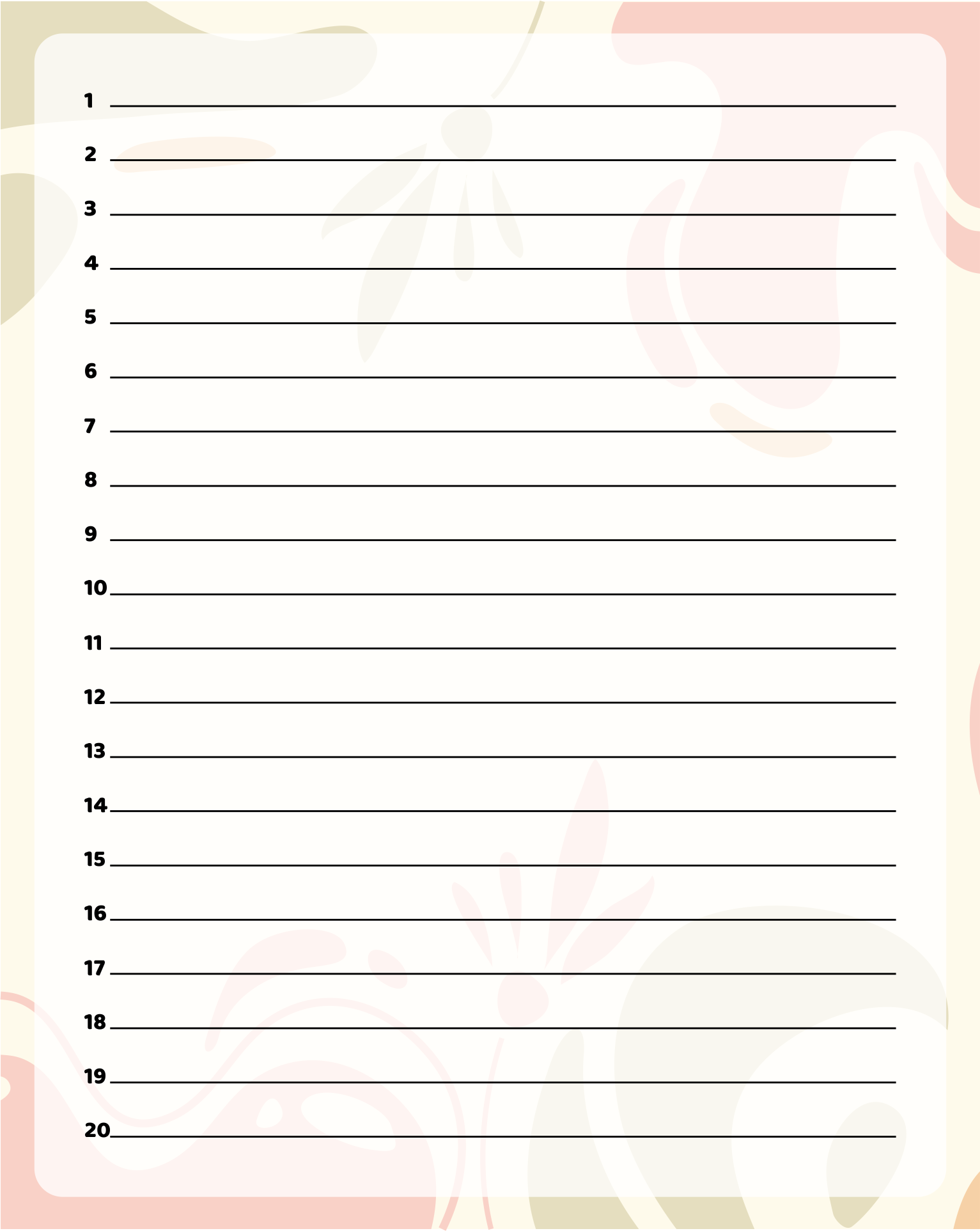### What are the uses of number lines in math?

Number lines are a valuable tool for teaching and learning math as they provide a clear and intuitive way for students to understand and work with numbers and mathematical concepts. There are several benefits to using number lines in learning math, including:

Number lines provide a visual representation of numbers and mathematical concepts, which can help students to better understand and retain information.

It is such a helpful tool to teach number sense, such as ordering and comparing numbers, as well as for solving problems involving fractions and decimals.

The number lines are great to introduce and reinforce mathematical operations such as addition, subtraction, multiplication, and division.

This is representing sequences of numbers which can help students to understand mathematical patterns and rules.

Number lines are able to help make the concept of negative numbers concrete and give meaning to the operations that can be performed with them.

If you need the tool to represent data, number lines are the exact option. It also can be used to teach concepts related to statistics and data analysis.

Number lines allow you to represent geometric and arithmetic sequences which can help students to understand the concepts of rates of change and common differences.

It's beneficial to students for developing problem-solving skills by providing a visual representation of the problem, which can make it easier for students to understand the problem and find a solution.

### How can I construct a number line?

When it comes to teaching math, number lines are included as a helpful tool. You might come up with this once to practice in your kids’ classroom. In order to have this, constructing by yourself is allowable. Here are a few steps to create number lines:

1. Decide on the range of numbers that you want to include on your number line. This could be a specific set of whole numbers, fractions, decimals, or even negative numbers.

2. Draw a straight line on a piece of paper or a whiteboard. This will be your number line. Mark the starting point of your number line. It could be zero, or another number depending on the range you have chosen.

3. Select the interval between tick marks. It is depending on the range of numbers you have chosen and the size of your number line. For example, if you are working with whole numbers, you might choose to have one tick mark for each whole number.

4. Mark tick marks along the number line at regular intervals, starting from the starting point. Label each of them with the corresponding number.

5. If the numbers you are working with are negative, you can extend the number line to the left of zero to include the negative numbers.

6. To make the number line more clear and easy to read, you can add labels to indicate the start and end of the number line, or you can use different colors to distinguish between positive and negative numbers.

7. Once the number line is complete, it can be used to represent numbers, sequences, and mathematical operations.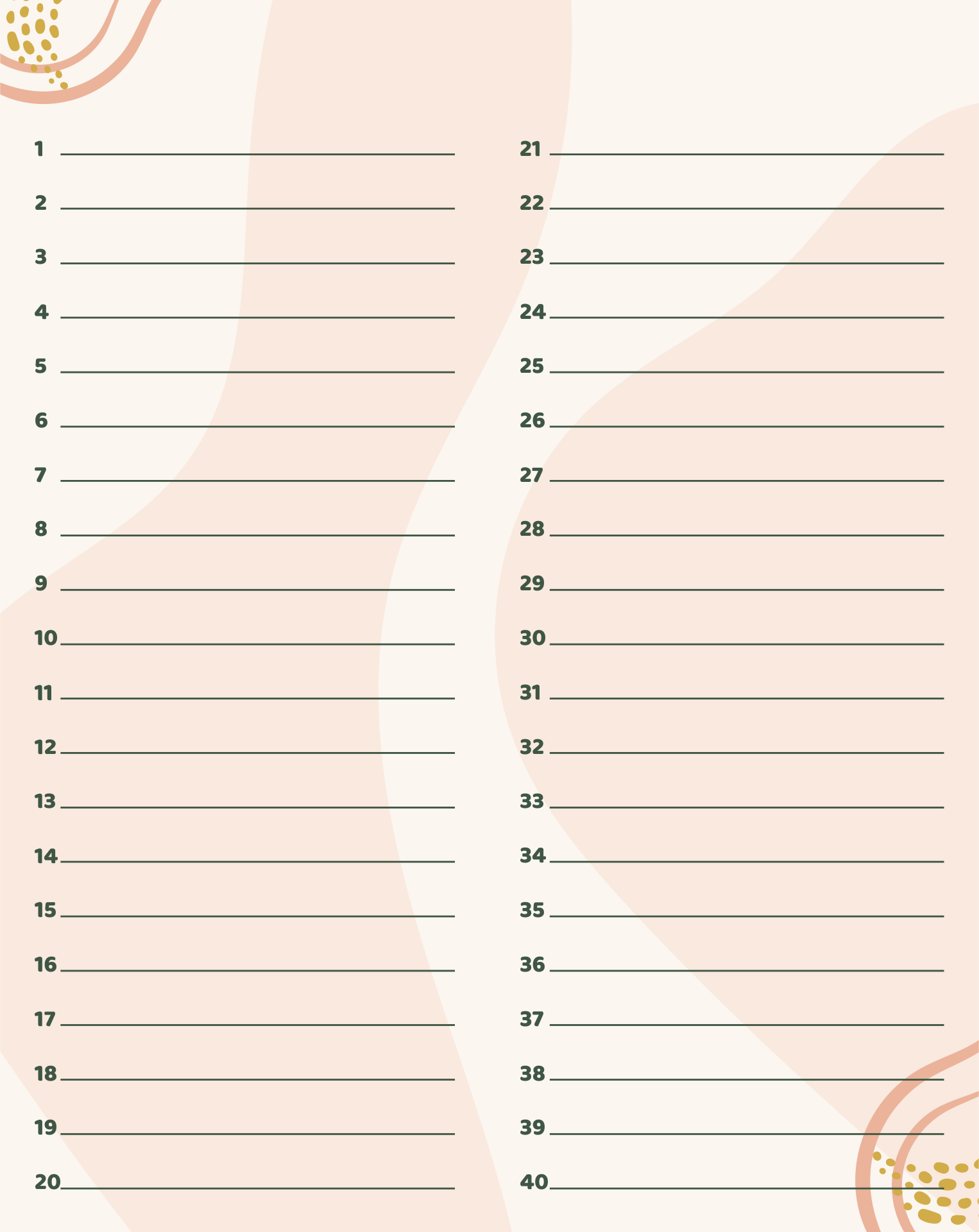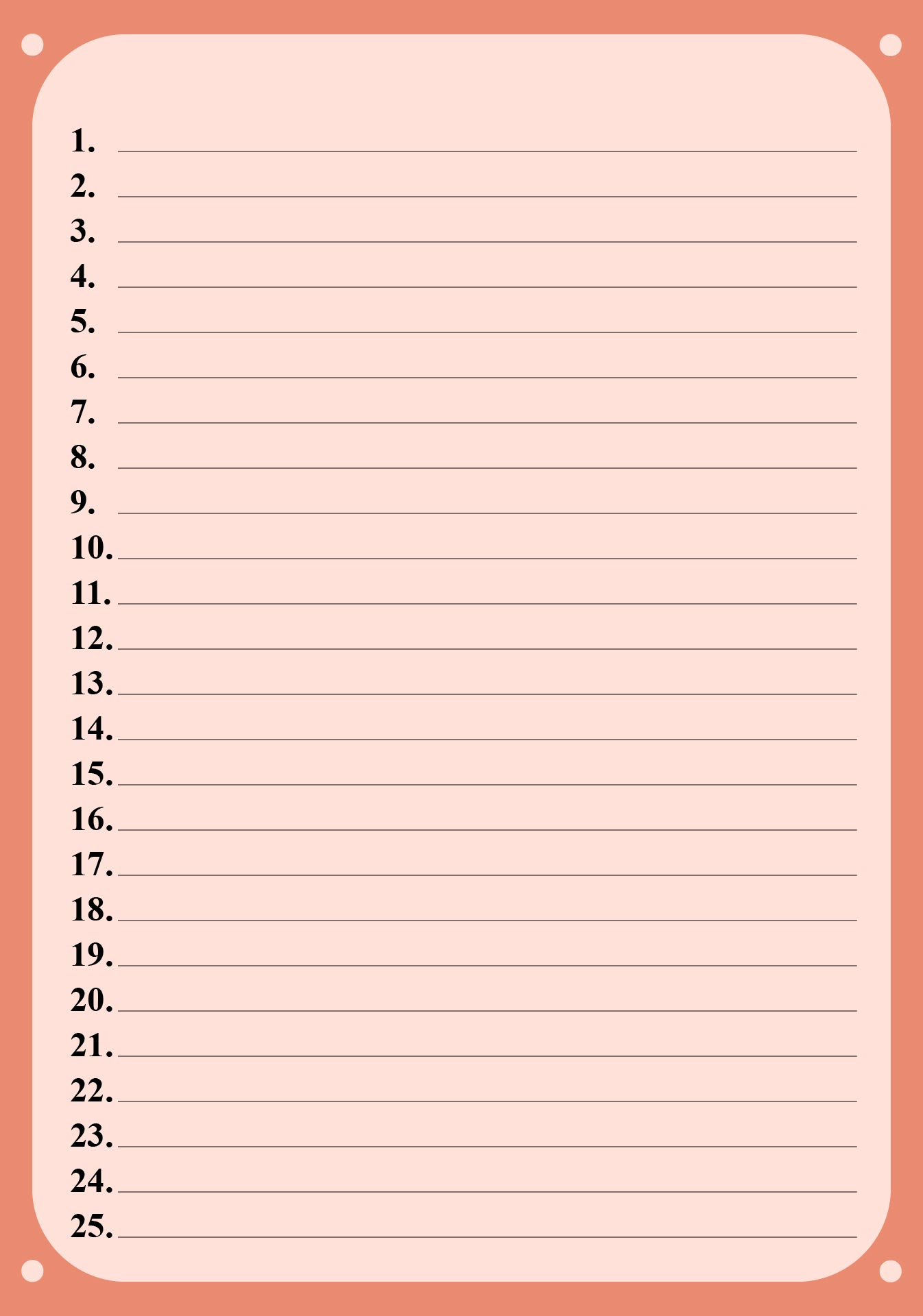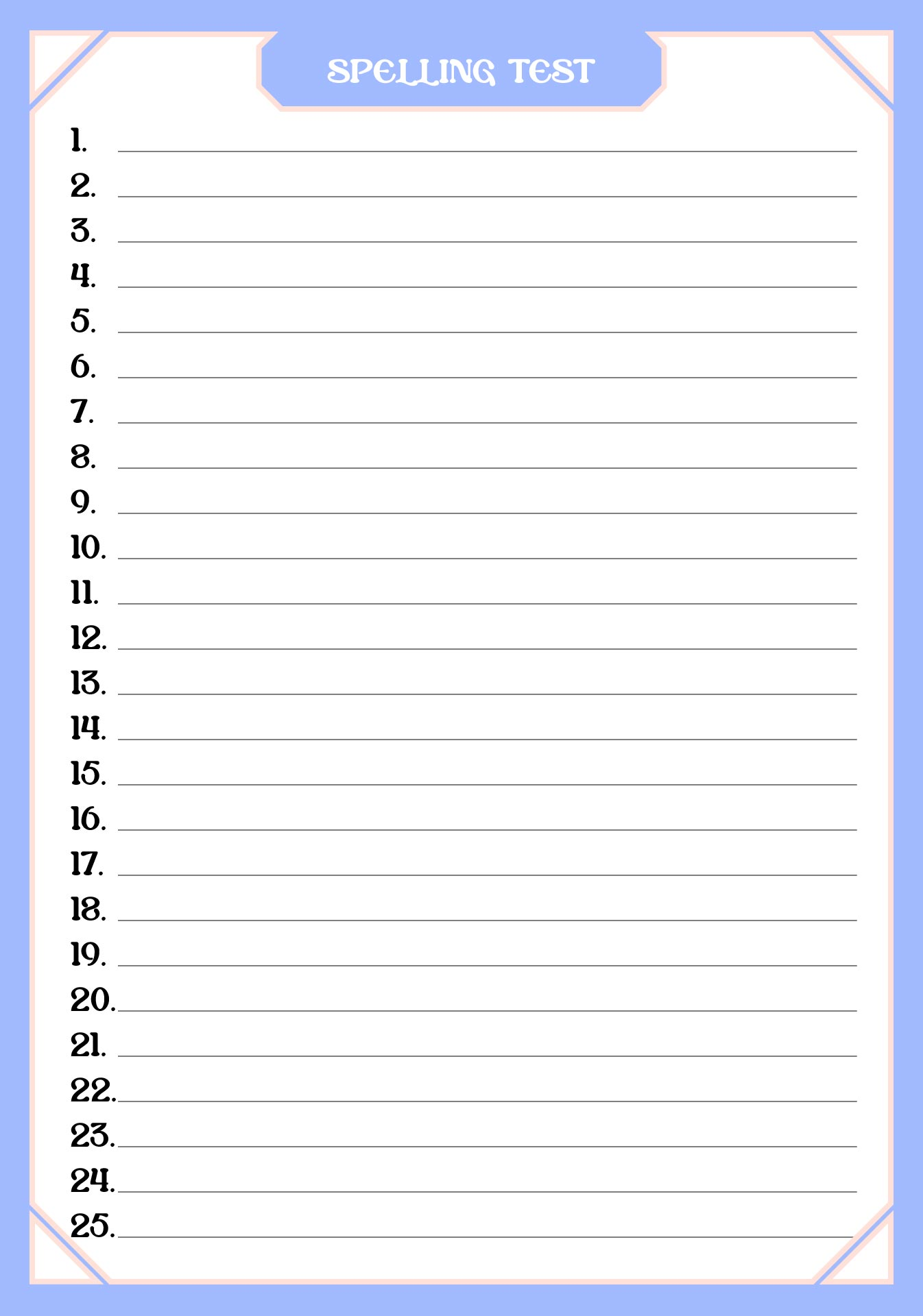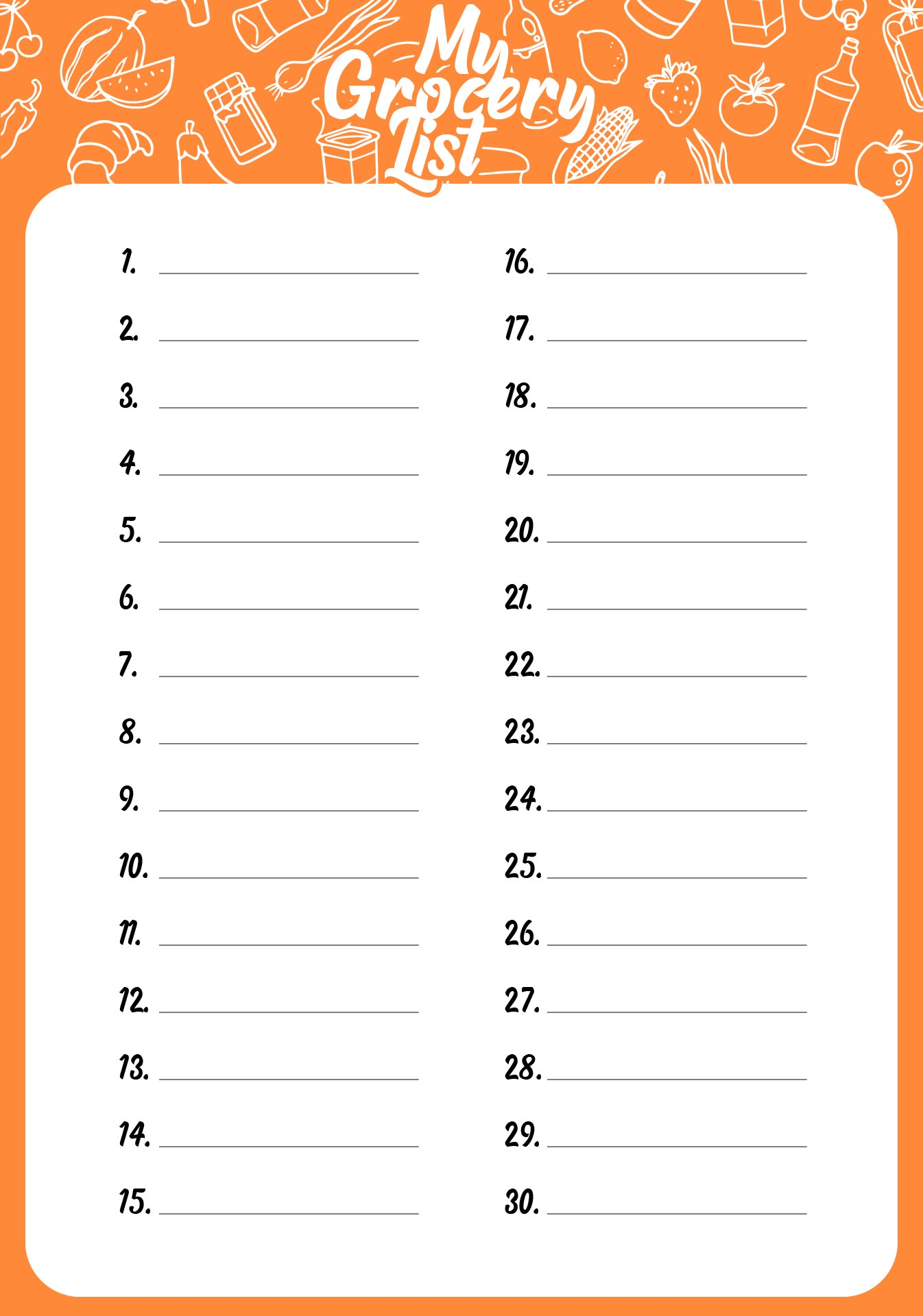### How many types of number lines are there?

Number lines are closely related to math learning. There are several types of number lines that are commonly used in mathematics education, including:

1. Whole number line

This type of number line is used to represent the set of whole numbers, including zero. The tick marks on this number line are placed at regular intervals, with one tick mark for each whole number.

1. Integer number line

This one is used to represent the set of integers, including positive and negative whole numbers. The tick marks on this number line are placed at regular intervals, with one tick mark for each integer.

1. Fraction number line

The fraction number line shows the set of fractions. The tick marks on this number line are placed at regular intervals to represent the fractions.

1. Decimal number line

It consists of decimal numbers and the tick mark placed at regular intervals to represent the decimal numbers.

1. Real number line

The real number lines consist of real numbers, which include all decimal numbers and irrational numbers.

1. Open number line

This type of number line is show numbers that are not whole numbers, such as decimals and fractions. The tick marks on this number line are located at intervals that are not whole numbers.

1. Temperature number line

It is used to present the temperature and allow you to use it in a more specific context like teaching physics or science.

1. Time number line

This number line presents the time and is great to use in custom fields such as teaching clock reading, time duration, and so on.

Different types of number lines are used to represent different types of numbers and mathematical concepts, and they can be used in different contexts depending on the needs of the students and the curriculum.